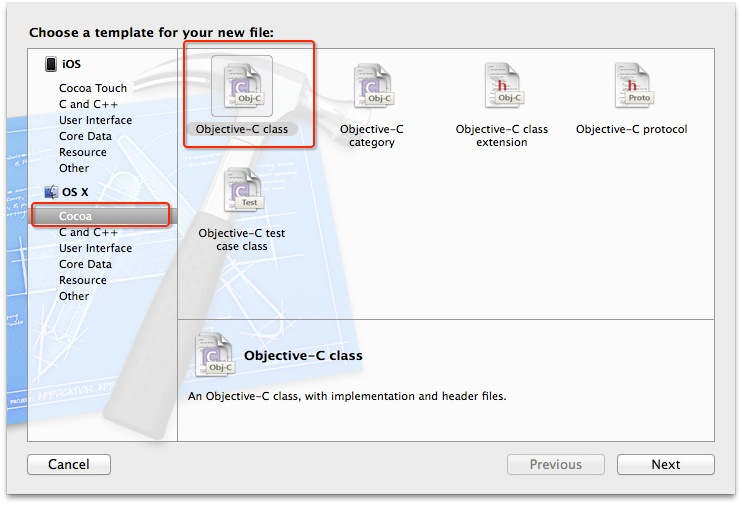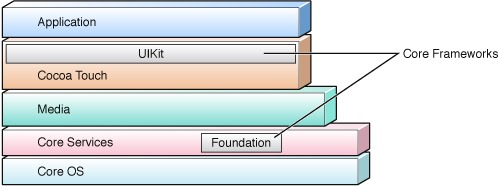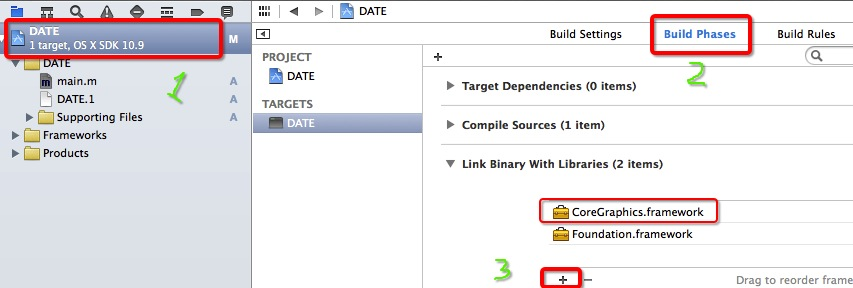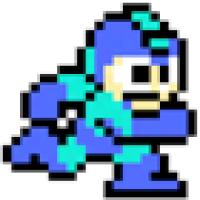结构体 原

Foundation框架概述Cocoa不是一种编程语言（它可以运行多种编程语言），它也不是一个开发工具（通过命令行我们仍然可以开发Cocoa程序），它是创建Mac OS X和IOS程序的原生面向对象API，为这两者应用提供了编程环境。Foundation中常用的结构体

sample code:

NSRange

#import <Foundation/Foundation.h>/*NSRange表示一个范围*/void test1(){
NSRange rg={3,5};//第一参数是起始位置第二个参数是长度
//NSRange rg;
//rg.location=3;
//rg.length=5;
//NSRange rg={.location=3,.length=5};
//常用下面的方式定义 NSRange rg2=NSMakeRange(3,5);//使用NSMakeRange定义一个NSRange
//打印NSRange可以使用Foundation中方法 NSLog(@"rg2 is %@", NSStringFromRange(rg2));//注意不能直接NSLog(@"rg2 is %@", rg2)，因为rg2不是对象（准确的说%@是指针）而是结构体}

/*rangeOfString*/
// 这个用来查找某一个字符串在整个字符串中得范围
// 如果找不到，length=0，location=NSNotFound==-1
NSString *str = @"i hate u!";
NSRange range = [str rangeOfString:@"u"];
NSLog(@"location is %ld,length is %ld",range.location,range.length);

输出结果是:location is 7,length is 1

NSPoint/CGPoint

/*NSPoint结构体是用来定义一个点x,y*/
// 一半常用的还是用CGPoint来定义点
NSPoint p1 = CGPointMake(1, 2);
// 输出的话是用字符串和关键字NSStringFromPoint
NSString *strPoint = (NSStringFromPoint(p1));
NSLog(@"%@",strPoint);

NSSize/CGSize

/*NSSize表示的是尺寸,宽和高*/
// 和NSRange一样,设置NSSize结构体也有两种方式,但我们还是记常用的这种方式
NSSize s1 = CGSizeMake(10, 20);
// 输出可以用字符串和关键字NSStringFromSize来接收
NSString *strSize = (NSStringFromSize(s1));
NSLog(@"%@",strSize);

NSRect/CGRect

/*NSRect可以理解为一NSPoint和NSSize的结合体*/
//我们不妨来看一下自定义一个NSRect的实际结构
CGRect myRect(CGFloat x, CGFloat y, CGFloat width, CGFloat height)
{
// 可以理解位CGRect里有(origin,size)
// origin里有两个点x和y,size里有宽和高width和height
CGRect rect;
rect.origin.x = x;
rect.origin.y = y;
rect.size.width = width;
rect.size.height = height;
return rect;
}
// 定义一个点是0,1.宽和高都是15的结构体r1
NSRect r1 = CGRectMake(0, 1, 15, 15);
NSString *strRect = (NSStringFromRect(r1));
NSLog(@"%@",strRect);

常用结构体总结

#import <Foundation/Foundation.h>
/*NSRange表示一个范围*/
void test1(){
NSRange rg={3,5};//第一参数是起始位置第二个参数是长度
//NSRange rg;
//rg.location=3;
//rg.length=5;
//NSRange rg={.location=3,.length=5};
//常用下面的方式定义 NSRange rg2=NSMakeRange(3,5);//使用NSMakeRange定义一个NSRange
//打印NSRange可以使用Foundation中方法 NSLog(@"rg2 is %@", NSStringFromRange(rg2));//注意不能直接NSLog(@"rg2 is %@", rg2)，因为rg2不是对象（准确的说%@是指针）而是结构体
NSLog(@"%@",NSStringFromRange(rg));
}
/*NSPoint表示一个点*/
void test2(){
NSPoint p2=NSMakePoint(10, 15);//NSPoint其实就是CGPoint
//这种方式比较常见 NSPoint p2=CGPointMake(10, 15);
NSLog(@"%@",NSStringFromPoint(p2));
}
/*NSSize表示大小*/
void test3(){
NSSize s2=NSMakeSize(10, 15);//NSSize其实就是CGSize
//这种方式比较常见 CGSize s2=CGSizeMake(10, 15);
NSLog(@"%@",NSStringFromSize(s2));
}
/*NSRect表示一个矩形*/
void test4(){
NSRect r2=NSMakeRect(10, 5, 100, 200);//NSRect其实就是CGRect
//这种方式比较常见 NSRect r2=CGRectMake(10, 5, 100, 200);
NSLog(@"%@",NSStringFromRect(r2));
}

int main(int argc, const char * argv[]) {
@autoreleasepool {
test1();
test2();
test3();
test4();
} return 0;
}

// 因为开发中经常用到(0,0)这个点
// 一般情况下就会用NSPoint p = CGPointMake(0,0);
// 但是开发中我们会用CGPointZero来表示原点

函数补充

#import <Foundation/Foundation.h>

int main()
{
/*CGRectEqualToRect*/
CGRect r1 = CGRectMake(10, 11, 20, 30);
// CGRectEqualToRect根据函数名可以知道这个函数是判断两个Rect结构体是否相等,返回类型是bool
bool b =  CGRectEqualToRect(CGRectMake(10, 11, 20, 30), r1); // 返回1
NSLog(@"%d",b1);

/*CGRectContainsPoint*/
// CGRectContainsPoint用来判断一个点是否在这个Rect范围内,返回值也是bool
bool b1 = CGRectContainsPoint(CGRectMake(0, 0, 30, 30), CGPointZero);
NSLog(@"%d",b1);

// 同样地,也有CGPointEqualToPoint和CGSizeEqualToSize这两个函数,都是用来判断的

return 0;
}Heisenbean

C语言-第八章、构造数据类型

8_1 结构体的概念和结构体变量 _1.1 结构体的概念 C语言中允许用户自己构造由不同数据类型的数据所组成的集合体，称为结构体。结构体属于数据类型，每一个结构体有一个名字，称为结构体名。一...

2016/11/05
31
0
Golang：结构体

2017/12/27
0
0
C语言基础-13-结构体

C语言的核心部分都说得七七八八了，相信大家已经对C语言的基本数据类型(charintfloat)、数组、指针都很熟悉了，今天来学习C语言中另外一种数据类型：结构体。在iOS开发中，结构体是经常用到的...

Corwien
2016/03/16
26
1
C语言结构体、枚举以及位域的讲解

2017/11/20
0
0

04/21
17
0

cleanLastUpdated.bat

@echo offrem create by AnXiaole rem 这里写你的仓库路径set REPOSITORY_PATH=C:\Users\AnXiaole\.m2\repositoryrem 正在搜索...for /f "delims=" %%i in ('dir /b /s "%REPO......

12分钟前
1
0

有区别 　　1、单级放大的倍数比较有限，一般在100倍以下。放大倍数很大的话，负反馈就比较浅，对于放大倍数的稳定性不利。假如需要放大倍数更高，就不得不动用多级放大电路了。单级放大...

15分钟前
2
0
c++ 上传文件 curl

bool uploadFile(std::string url, std::string file, std::string auth) { boost::filesystem::path p(file); CURL *curl; CURLcode res; struct curl_httppost *for......

21分钟前
2
0

// 冒泡排序，a 表示数组，n 表示数组大小public void bubbleSort(int[] a, int n) { if (n <= 1) return; for (int i = 0; i < n; ++i) { // 提前退出冒泡循环的标志位 ...

23分钟前
3
0
centos7.6 +mhvtl1.6安装

25分钟前
2
0